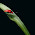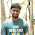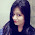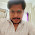VHDL coding tips and tricks: Using real data types in VHDL

## Thursday, June 16, 2011

### Using real data types in VHDL

Apart from the standard types like integer and std_logic_vector's VHDL also offer real data types. But a real data type has a big disadvantage. It is not synthesis-able. It can be used only for simulation purposes. This disadvantage limits its use to a large extend, but there are plenty of projects where we look only for simulation results.

Before starting the coding part of a VHDL project,one has to decide whether the project to be implemented on a real FPGA or just a computer simulation is required. If it has to be ran on FPGA, then forget about the real package and use only synthesis-able data types like std_logic,integer etc... Otherwise you can reduce the time and complexity of your project by using real data types.

The real data type is defined in the library called MATH_REAL. So you have to include the following line before the entity declaration in the code:
use ieee.math_real.all;

The math_real package also offers some elementary mathematical functions for real data types. You can see the math_real.vhd file at the following address.

See the below code to get an idea on how to use these functions:

library IEEE;
use IEEE.STD_LOGIC_1164.ALL;
use IEEE.MATH_REAL.ALL;

entity real_demo is
end real_demo;

architecture Behavioral of real_demo is

--signals declared with the REAL data type.
--MATH_PI is a constant defined in the math_real package.
signal X : real := -MATH_PI/3.0; --A real variable X, initialized to pi/3(60 degreee).
signal sign_result,ceil_result,floor_result,round_result,trunc_result : real := 0.0;
signal max,min,root,cube,power1,power2,exp_result : real := 0.0;
signal log_result,log2_result,log10_result,log_result2,sine,cosine,tangent : real := 0.0;
signal sin_inv,cos_inv,tan_inv,sin_hyp,cos_hyp,tan_hyp : real := 0.0;
signal inv_sin_hyp,inv_cos_hyp,inv_tan_hyp : real := 0.0;

begin

process
begin

sign_result <= SIGN(X);  --sign of X
ceil_result <= CEIL(X); --smallest integer value not less than X
floor_result <= FLOOR(X); --largest integer value not greater than X
round_result <= ROUND(X); --round to the nearest integer.
trunc_result <= TRUNC(X); --truncation.
max <= REALMAX(4.5,4.6); --return the maximum
min <= REALMIN(2.3,3.2); --return the minimum
root <= SQRT(4.0);  --square root
cube <= CBRT(64.0); --cube root
power1 <= 2**3.0; --power of an integer
power2 <= 3.0**3.0; --power of a real
exp_result <= EXP(1.0); --returns e**X.
log_result <= LOG(2.73); --natural logarithm
log2_result <= LOG2(16.0); --log to the base 2.
log10_result <= LOG10(100.0); --log to the base 10.
log_result2 <= LOG(27.0,3.0); --log to the given base.
sine <= SIN(X); --sine of the given angle(in rad)
cosine <= COS(X);--cosine of the given angle(in rad)
tangent <= TAN(X);--tangent of the given angle(in rad)
sin_inv <= ARCSIN(SIN(X)); --sine inverse.
cos_inv <= ARCCOS(COS(X)); --cosine inverse.
tan_inv <= ARCTAN(TAN(X)); --tangent inverse.
sin_hyp <= SINH(X); --Hyperbolic sine
cos_hyp <= COSH(X); --Hyperbolic cosine.
tan_hyp <= TANH(X); --Hyperbolic tangent.
inv_sin_hyp <= ARCSINH(SINH(X)); --Inverse hyperbolic sine.
inv_cos_hyp <= ARCCOSH(COSH(X)); --Inverse hyperbolic cosine.
inv_tan_hyp <= ARCTANH(TANH(X)); --Inverse hyperbolic tangent.
wait;

end process;

end Behavioral;

Almost all the functions available in the real package are shown above and comments are provided on what they are used for. For your reference I have attached the simulation result below:

As you can see its a pretty useful library. Dont forget to use it if you have a simulation project in VHDL. Contact me for any kind of help. I always use this package when I need to convert a matlab code to corresponding VHDL(for just simulation). Using this package, helps me to write the vhdl code like a pseudo code.

#### 9 comments:

1.Hi,
I've error of "type real not supported in xilinx". your post was useful, but I need to know clearly that to solve that problem I need to download the "math_real.vhd file" from the give address.
where do I need to save it ?

Kindly give suggestions

Thank you,

Arunaa

2.@Arunaa : you dont need to download the file from the given address. The address is given for just your reference.Include the libraries properly and it shouldnt give any error. But it wont work in synthesis as i mentioned in the post.
If you are still getting the error, try using the latest version of xilinx.

3.X0 : in integer range 1 to 255 ;
Y0 : out integer range 1 to 500 ;
and i use:
Y0 <= natural(ceil(real(255)/real(X0)));
the result error.
i hope everyone help me to repair error it

4.Out of interest, if I need these functions as part of a generate statement for an algorithm which dynamically creates structures based on generics however don't use any Real types in the design behavior itself, would that work and how?

Hopefully someone knows, thanks :-)

5.hello, I also had errors when compiling, someone can tell me why

6.how can i give the inputs using a testbench. pls help me out with a testbench code.

7.If anyone knows ans plz send me reply on my I'd -soni21sin@gmail.com. Thnx for help

8.If i generate sine wave in modelsim using VHDL code then only +ve wave is shown on the waveform. Can anyone help me why -ve value is not generated.

9.will it work for negative values also ?????